• +91 9971497814
• info@interviewmaterial.com

# Polynomials Ex-2.1 Interview Questions Answers

### Related Subjects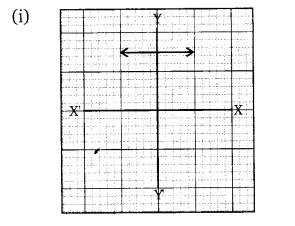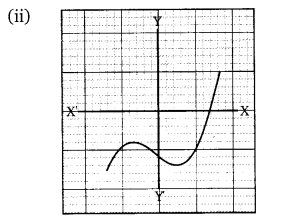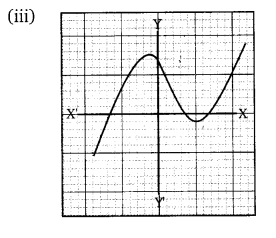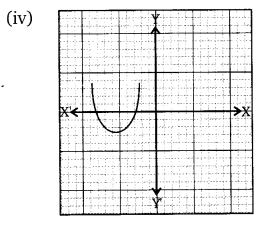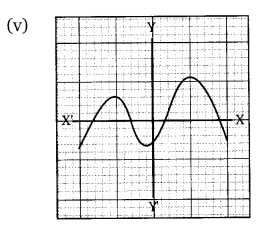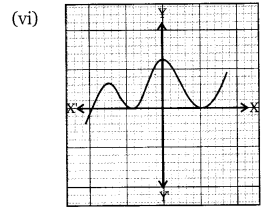Note  :-  Total number of zeroes in any polynomial equation = total numberof times the curve intersects x-axis.

(i) In the given graph, the number of zeroesof p(x) is 0 because the graph is parallel to x-axis does not cut it at anypoint.

(ii) In the given graph, the number of zeroesof p(x) is 1 because the graph intersects the x-axis at only one point.

(iii) In the given graph, the number of zeroesof p(x) is 3 because the graph intersects the x-axis at any three points.

(iv) In the given graph, the number of zeroesof p(x) is 2 because the graph intersects the x-axis at two points.

(v) In the given graph, the number of zeroesof p(x) is 4 because the graph intersects the x-axis at four points.

(vi) In the given graph,the number of zeroes of p(x) is 3 because the graph intersects the x-axis atthree points.

Todays Deals### Polynomials Ex-2.1 Contributorskrishan

Name:
Email:

# Latest News# 9000 interview questions in different categories